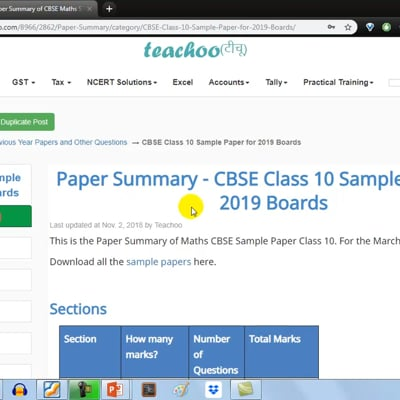CBSE Class 10 Sample Paper for 2019 Boards

Class 10
Solutions of Sample Papers for Class 10 Boards

This is the Paper Summary of Maths CBSE Sample Paper Class 10. For the March 2019 Board Exams.This video is only available for Teachoo black users

## Sections

 Section How many marks? Number of Questions Total Marks Section A 1 marks each 6 1 × 6 = 6 Section B 2 marks each 6 2 × 6 = 12 Section C 3 marks each 10 3 × 10 = 30 Section D 4 marks each 8 4 × 8 = 32 Total 30 questions 80 marks

Total marks is 100, out which 20 marks is Internal Assesment - which is sent by your school to CBSE

## Marks per Chapter

According to CBSE Syllabus, the marks per unit is like this

 Units Chapters of NCERT Marks I. Number System Chapter 1 6 II. Algebra Chapter 2, 3, 4, 5 20 III. Coordinate Geometry Chapter 7 6 IV. Geometry Chapter 6, 10, 11 15 V. Trigonometry Chapter 8, 9 12 VI. Mensuration Chapter 12, 13 10 VII. Statistics & Probability Chapter 14, 15 11 Total 80

In this sample paper,

marks per chapter is

 Chapter 1 Mark Questions 2 Mark Questions 3 Mark Questions 4 Mark Questions Total Marks Chapter 1 - Real Numbers 1 1 OR Question 1 6 Chapter 2 - Polynomials 1 3 Chapter 3 - Pair of Linear Equations in Two Variables 1 1 5 Chapter 4 - Quadratic Equations 1 OR Question 1 OR Question 5 Chapter 5 - Arithmetic Progressions (AP) 1 1 OR Question 1 7 Chapter 6 - Triangles 1 1 OR Question 1 Theorem 8 Chapter 7 - Coordinate Geometry 1 1 1 OR Question 6 Chapter 8 - Trigonometry 1 OR Question 1 OR Question 1 8 Chapter 9 - Applications of Trigonometry (Heights and Distances) 1 OR Question 4 Chapter 10 - Circles 1 3 Chapter 11 - Constructions 1 4 Chapter 12 - Areas related to circle 1 3 Chapter 13 - Surface Area and Volume 1 OR Question 1 7 Chapter 14 - Statistics 1 1 OR Question 7 Chapter 15 - Probability 2 4 Total 80

This marks division, as well as number of questions per section is exactly the same as last year.

There are total 2 + 2 + 4 + 3 = 11 OR Questions.

## Observations from the paper

• Some 1 mark questions have step marking

• When cancelling square roots, don't forget to write plus-minus sign ±

• Writing units is important or half marks is cut, eg: x =3 is wrong, x = 3 metre is right.

• Formula of all chapters has 1/2 marks

• Formula of Statistics (Chapter 14) has 1 mark

• In Chapter 9 Heights and Distances, 1 mark is given for correct figure

• 1 Question of Theorem will come. (Of Chapter 6 or 10)

• 1 4-Marks question of Construction will come

• Remember the formula for Length of an arc (Chapter 12)

Length of arc = θ/360 × 2πr
It is used when length of arc is given, and we need to find angle

• NCERT Questions are very important  - 3 Questions out of 30 have come straight from NCERT. Other questions is very similar

• In questions where the answer can be found easily (like finding curved surface area of frustum if height and radius is given), calculation is difficult. 1 mark will be deducted if you have not found the exact answer

• All questions of the Sample Paper has been solved, with marking scheme also given of each and every step. Click next to start doing the questions

• Question 30 is tricky. Please check it.

## Tips for CBSE Boards 2019

• Do the chapters from the Concept wise. Check out the video below
• Remember all the Theorems and their proofs.

• Remember all formulas of all Chapters. Formula sheet is provided for all chapters

• Important questions have been marked in all chapters. Check them while revising

• It is important to Revise the topics from Concept wise . Just check the theory, and the questions in order they are given

• Make sure you have 2 pencils, 1 compass, scale, eraser, sharpener with you in the geometry box
• Make sure you do atleast this year's sample paper.

• Do not miss any steps. Write each and every step possible.

Have anything else? Comment below to tell me.

All the best!

Solve all your doubts with Teachoo Black (new monthly pack available now!)